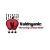true

Take Class 12 Tuition from the Best Tutors

•Affordable fees
•1-1 or Group class
•Flexible Timings
•Verified Tutors

Search in

# Having trouble using Kinematic Equations?Shuddhodan Sapre
11/04/2021 00

How to use kinematic equations and when to use them?

We all know the 3 kinematic equations which are

S= ut + 1/2 at²

V = u + at

V²= u² + 2as

Many students find it difficult to understand when to use them and how to use them.

Just keep a watch for the following points:

1) The equations are used only when acceleration is constant or zero. So while reading a question check whether a body has constant acceleration which will be directly mentioned in the question or will be indirectly given by saying something like " a constant force acts on the body " (which means constant acceleration since F=ma) or "velocity is constant" ( this means acceleration is zero.

2) If you observe these equations have 5 terms u,v,a,t and S. So many a times when quantities like velocity or acceleration are required for calculating quantities like kinetic energy ( = 1/2 mv²) or Force (=ma) they will give you the other terms. For example lets say you are asked final kinetic energy, to calculate that you will require mass and final velocity (V) but instead of velocity the question has stated the body starts from rest which means initial velocity (u)  is zero and time t is given along with acceleration a. By using v=u+at you can easily calculate v and hence Kinetic Energy.

So no matter what the chapter is find atleast 3 terms out of the 5 terms of the equations if you spot them that is the biggest hint for using kinematic equations.

Note : These won't be used in cases where acceleration changes.

0 Dislike
Follow 1## Other Lessons for You

How to feel conservation of momentum practically ?
Lets say you are standing on a frictionless platform of ice. And you are holding a 10kg bag . You cannot walk on this platform as there is no frction , you will slide. Now lets say you want to move in...Electric field
ELECTRIC FIELD : The space or region around the charge in which its effect can be felt is called electric field. Electric field is denoted by 'E'. In other words, if we...
D

Importance of Bouncing in Collisions
There are 3 Types of Collisions Elastic, Inelastic and Totally Inelastic collision. Momentum is conserved in all these three types ( No external force is involved, so the system is called a Closed System....Law of conservation of Linear momentum
According th o this law if net force acting on an isolated system is zero then linear momentum remains conserved. i.e. momentum just before the instant considered is equal to momentum after. But we...### Looking for Class 12 Tuition ?

Learn from Best Tutors on UrbanPro.

Are you a Tutor or Training Institute?

Join UrbanPro Today to find students near you
X

### Looking for Class 12 Tuition Classes?

The best tutors for Class 12 Tuition Classes are on UrbanPro

• Select the best Tutor
• Book & Attend a Free Demo
• Pay and start Learning### Take Class 12 Tuition with the Best Tutors

The best Tutors for Class 12 Tuition Classes are on UrbanPro